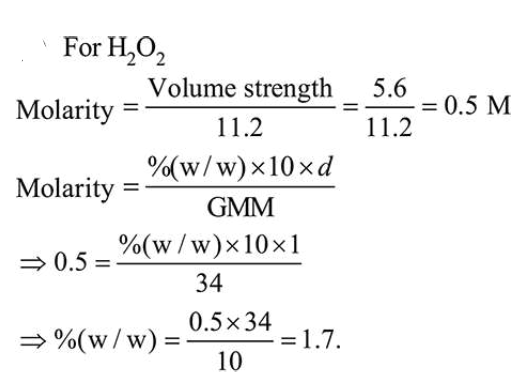# The strengths of 5.6 volume hydrogen peroxideQuestion:

The strengths of $5.6$ volume hydrogen peroxide (of density $1 \mathrm{~g} / \mathrm{mL})$ in terms of mass percentage and molarity $(\mathrm{M})$, respectively, are:

(Take molar mass of hydrogen peroxide as $34 \mathrm{~g} / \mathrm{mol}$ )

1. $1.7$ and $0.5$

2. $0.85$ and $0.25$

3. $1.7$ and $0.25$

4. $0.85$ and $0.5$

Correct Option: 1

Solution: